NEET  >  Test: Mechanical Properties of Solids

# Test: Mechanical Properties of Solids

Test Description

## 30 Questions MCQ Test Physics Class 11 | Test: Mechanical Properties of Solids

Test: Mechanical Properties of Solids for NEET 2023 is part of Physics Class 11 preparation. The Test: Mechanical Properties of Solids questions and answers have been prepared according to the NEET exam syllabus.The Test: Mechanical Properties of Solids MCQs are made for NEET 2023 Exam. Find important definitions, questions, notes, meanings, examples, exercises, MCQs and online tests for Test: Mechanical Properties of Solids below.
Solutions of Test: Mechanical Properties of Solids questions in English are available as part of our Physics Class 11 for NEET & Test: Mechanical Properties of Solids solutions in Hindi for Physics Class 11 course. Download more important topics, notes, lectures and mock test series for NEET Exam by signing up for free. Attempt Test: Mechanical Properties of Solids | 30 questions in 30 minutes | Mock test for NEET preparation | Free important questions MCQ to study Physics Class 11 for NEET Exam | Download free PDF with solutions
 1 Crore+ students have signed up on EduRev. Have you?
Test: Mechanical Properties of Solids - Question 1

### A perfectly rigid body is one

Detailed Solution for Test: Mechanical Properties of Solids - Question 1

Explanation:A perfectly rigid body is hypothetical in nature but for some phenomena ( in rotational bodies ) we assume bodies are perfectly rigid i.e. the intermolecular forces are always in equilibrium irrespective of the external forces due to which their shape and size are constant.

Test: Mechanical Properties of Solids - Question 2

### A steel rod 2.0 m long has a cross-sectional area of 0.30 cm2 . It is hung by one end from a support, and a 550-kg milling machine is hung from its other end. Determine the resulting strain. Young's Modulus of Steel (Y) = 20×1010 Pa

Detailed Solution for Test: Mechanical Properties of Solids - Question 2

Stress = F⟂/A = (Mass x Acceleration⟂)/Cross-sectional Area

= (550 kg x 9.8 m/s2)/ (0.30×10−4 m2)

= 1.8×108 Pa

Young's ModulusSteel (Y) = 20×1010 Pa = Stress/Strain

=> Strain = Stress/Y = 1.8×108 Pa / 20×1010 Pa

= 9.0×10−4

Test: Mechanical Properties of Solids - Question 3

### A piece of copper having a rectangular cross-section of 15.2 mm × 19.1 mm is pulled in tension with 44,500 N force, producing only elastic deformation. Calculate the resulting strain? Take Young's modulus of copper as 11 × 1010Pa

Detailed Solution for Test: Mechanical Properties of Solids - Question 3

Given Data,
Length of the piece of copper = l = 19.1 mm = 19.1 × 10-3m
Breadth of the piece of copper = b = 15.2 mm = 15.2× 10-3m
Tension force applied on the piece of cooper, F = 44500N
Area of rectangular cross section of copper piece,
Area = l× b
⇒ Area = (19.1 × 10-3m) × (15.2× 10-3m)
⇒ Area = 2.9 × 10-4 m2
Modulus of elasticity of copper from standard list, η = 42× 109 N/m2
By definition, Modulus of elasticity, η = stress/strain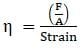⇒ Strain = F/Aη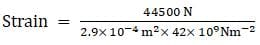⇒ Strain = 3.65 × 10-3
Hence, the resulting strain is 3.65 × 10-3

Test: Mechanical Properties of Solids - Question 4

What diameter should a 10-m-long steel wire have if we do not want it to stretch more than 0.5 cm under a tension of 940 N? Take Young's modulus of steel as 20 × 1010 Pa

Detailed Solution for Test: Mechanical Properties of Solids - Question 4

Y=F x l/A x Δ l
Δ l=0.5cm=0.5x10-2m, l=10M, F=940N
Y=20x1010pa
20x1010=940x10/πr2x0.5x10-10
πr2=94x100/5x10-3x2x1011=94x102/10x108
r2=94/π x 10-7 =2.99 x 10-6
r2 ≅3x10-6
r=1.13x10-10 m
diameter=2r=3.6mm

Test: Mechanical Properties of Solids - Question 5

Elasticity is the property of a body, by virtue of which

Detailed Solution for Test: Mechanical Properties of Solids - Question 5

Explanation:When external force is applied on the solid bodies, the solid bodies get deformed. The atoms or molecules are displaced from their equilibrium positions causing a change in the interatomic ( or intermolicular ) distances. When the deforming foce is removed, the interatomic forces tend to drive them back to their original postions. Thus the body regains its original shape and size.

Test: Mechanical Properties of Solids - Question 6

Elastomers are materials

Detailed Solution for Test: Mechanical Properties of Solids - Question 6

An elastomer is a polymer with viscoelasticity (i. e., both viscosity and elasticity) and very weak intermolecular forces, and generally low Young's modulus and high failure strain compared with other materials. Elastomer rubber compounds are made from five to ten ingredients, each ingredient playing a specific role. Polymer is the main component, and determines heat and chemical resistance, as well as low- temperature performance. Reinforcing filler is used, typically carbon black, for strength properties.

Test: Mechanical Properties of Solids - Question 7

A steel rod 2.0 m long has a cross-sectional area of 0.30 cm2. It is hung by one end from a support, and a 550-kg milling machine is hung from its other end. Determine the elongation. Take Young's modulus of steel as 20 × 1010 Pa

Detailed Solution for Test: Mechanical Properties of Solids - Question 7

σ=Stress and ε=strain
σ=F/A= (550kg) × (9.81m/s2)3×10-5m2/=0.18GPA
ε=Δl/l0=σ/Υ​=0.18×109/200×109​=9×10-4
Δl=εl0= (9×10-4) (2m) = 0.0018m=1.8mm

Test: Mechanical Properties of Solids - Question 8

A 200-kg load is hung on a wire with a length of 4.00 m, a cross-sectional area of 0.200 × 10−4 m2, and a Young’s modulus of 8.00 × 1010N/ m2. What is its increase in length?

Detailed Solution for Test: Mechanical Properties of Solids - Question 8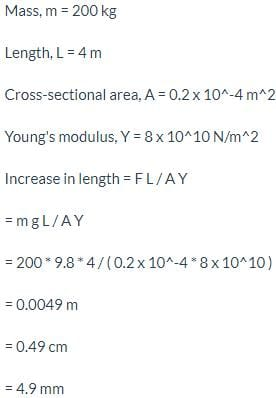Test: Mechanical Properties of Solids - Question 9

The importance of the elastic behavior of materials is

Detailed Solution for Test: Mechanical Properties of Solids - Question 9

Explanation:More the elastic a material is , more it has the property to regain its original position which is required in construction works.

Test: Mechanical Properties of Solids - Question 10

Shear modulus or modulus of rigidity is

Detailed Solution for Test: Mechanical Properties of Solids - Question 10

Shear modulus or modulus of rigidity is the ratio of shearing stress to the corresponding shearing strain, by the definition of shear modulus.

Test: Mechanical Properties of Solids - Question 11

Compute the fractional change in volume of a glass slab, when subjected to a hydraulic pressure of 10 atm. Bulk modulus of glass 37 GPa.

Test: Mechanical Properties of Solids - Question 12

If the elastic limit of copper is 1.5 × 108 N/ m2, determine the minimum diameter a copper wire can have under a load of 10.0 kg if its elastic limit is not to be exceeded.

Detailed Solution for Test: Mechanical Properties of Solids - Question 12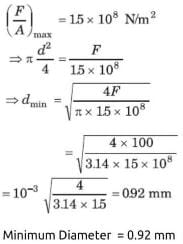Test: Mechanical Properties of Solids - Question 13

When a solid is deformed,

Detailed Solution for Test: Mechanical Properties of Solids - Question 13

Explanation:External force permanently distubed the equilibrium position of the interatomic ( or intermolecular ) forces between the particles of solid bodies.

Test: Mechanical Properties of Solids - Question 14

Rectangular section is rarely used in beams because

Detailed Solution for Test: Mechanical Properties of Solids - Question 14

I section is generally used as a beam because of its high section modulus as its most of the area is situated away from its neutral axis hence it has high moment of inertia i.e. high section modulus i.e. high moment carrying capacity which is the major requirement for a good beam section.

Test: Mechanical Properties of Solids - Question 15

A circular steel wire 2.00 m long must stretch no more than 0.25 cm when a tensile force of 400 N is applied to each end of the wire. What minimum diameter is required for the wire?

Detailed Solution for Test: Mechanical Properties of Solids - Question 15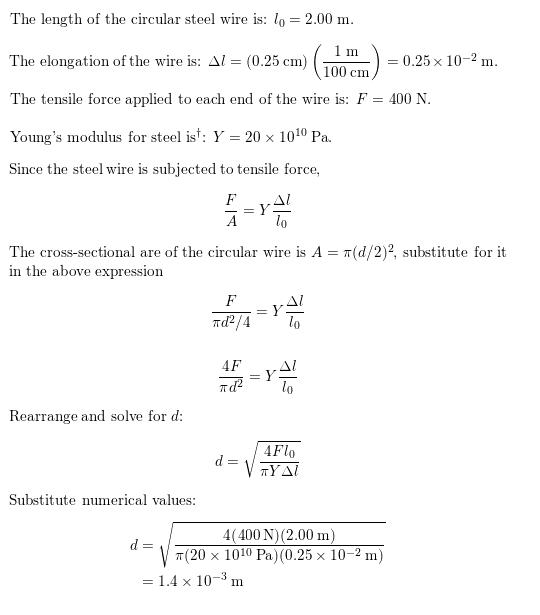Test: Mechanical Properties of Solids - Question 16

A mild steel wire of length 1.0 m and cross-sectional area 0.50 × 10−2 cm2 is stretched, well within its elastic limit, horizontally between two pillars. A mass of 100 g is suspended from the mid-point of the wire. Calculate the depression at the midpoint.

Detailed Solution for Test: Mechanical Properties of Solids - Question 16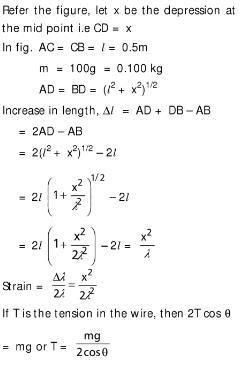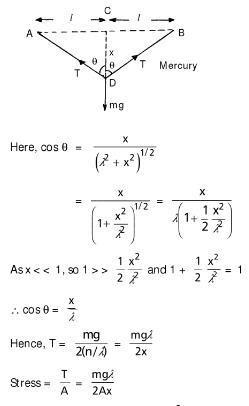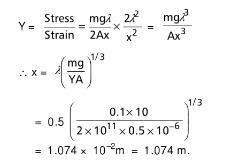Test: Mechanical Properties of Solids - Question 17

You hang a flood lamp from the end of a vertical steel wire. The flood lamp stretches the wire 0.18 mm and the stress is proportional to the strain. How much would it have stretched if the wire were twice as long?

Detailed Solution for Test: Mechanical Properties of Solids - Question 17

F/A = e/L
F/A = 0.18/l ( let the length be l)
F/A = x/2l
0.18/L = x/2l
0.18×2l = xl
0.36l = xl
x = 0.36

Test: Mechanical Properties of Solids - Question 18

Stress is

Test: Mechanical Properties of Solids - Question 19

Detailed Solution for Test: Mechanical Properties of Solids - Question 19

Explanation : The load applied to a column would place the column in compression; conversely, a load hanging from a rod would place the rod in tension. Strain is the deformation of a structural member because of stress within the member.

Test: Mechanical Properties of Solids - Question 20

The S.I unit of stress is

Detailed Solution for Test: Mechanical Properties of Solids - Question 20

Stress has its own SI unit called the Pascal. 1 Pascal (Pa) is equal to 1 N/m2. In imperial units stress is measured in pound force per square inch which is often shortened to "psi". The dimension of stress is same as that of pressure.

Test: Mechanical Properties of Solids - Question 21

Material is said to be ductile if

Detailed Solution for Test: Mechanical Properties of Solids - Question 21

A ductile material is one that can withstand a large amount of plastic deformation between the elastic limit and the fracture point.
A material that breaks suddenly when elongated or fracture occurs in it soon after the elastic limit is crossed is called a brittle material.
A ductile material that exhibits extra elongation or deformation and does not fracture is also referred as superplastic material.

Test: Mechanical Properties of Solids - Question 22

In constructing a large mobile, an artist hangs an aluminum sphere of mass 6.0 kg from a vertical steel wire 0.50 m long and 2.5 × 10−3 cm2in cross-sectional area. On the bottom of the sphere he attaches a similar steel wire, from which he hangs a brass cube of mass 10.0 kg. Compute the elongation.

Detailed Solution for Test: Mechanical Properties of Solids - Question 22

Hence 1.6 mm upper, 1.0 mm lower is correct.

Test: Mechanical Properties of Solids - Question 23

volume strain is defined

Test: Mechanical Properties of Solids - Question 24

A steel wire of length 4.7 m and cross-sectional area 3.0 × 10−5 m2 stretches by the same amount as a copper wire of length 3.5 m and cross-sectional area of 4.0 × 10−5 m2 under a given load. What is the ratio of the Young’s modulus of steel to that of copper?

Detailed Solution for Test: Mechanical Properties of Solids - Question 24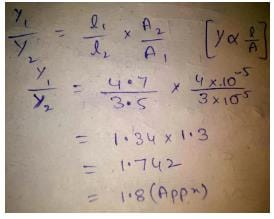Test: Mechanical Properties of Solids - Question 25

A lead cube measures 6.00 cm on each side. The bottom face is held in place by very strong glue to a flat horizontal surface, while a horizontal force F is applied to the upper face parallel to one of the edges. How large must F be to cause the cube to deform by 0.250 mm? (Shear modulus of lead = 0.6 × 1010Pa)

Detailed Solution for Test: Mechanical Properties of Solids - Question 25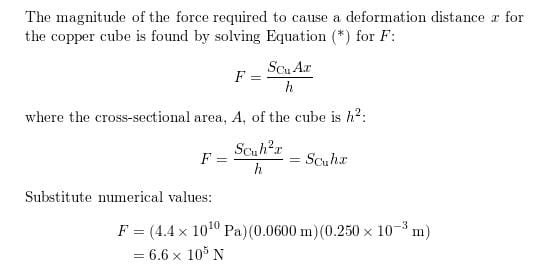Test: Mechanical Properties of Solids - Question 26

Two strips of metal are riveted together at their ends by four rivets, each of diameter 6.0 mm. What is the maximum tension that can be exerted by the riveted strip if the shearing stress on the rivet is not to exceed 6.9 × 107Pa? Assume that each rivet is to carry one quarter of the load.

Detailed Solution for Test: Mechanical Properties of Solids - Question 26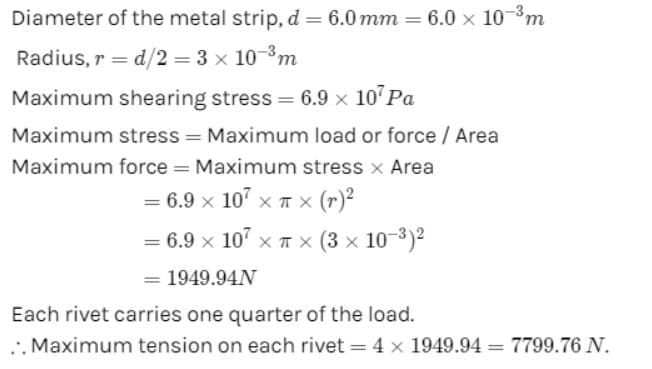Test: Mechanical Properties of Solids - Question 27

According to Hooke’s law

Detailed Solution for Test: Mechanical Properties of Solids - Question 27

Hook’s Law states that for small deformations the stress and strain are proportional to each other.

Test: Mechanical Properties of Solids - Question 28

You hang a flood lamp from the end of a vertical steel wire. The flood lamp stretches the wire 0.18 mm and the stress is proportional to the strain. How much would it have stretched if the wire had the same length but twice the diameter?

Test: Mechanical Properties of Solids - Question 29

With reference to figure the elastic zone is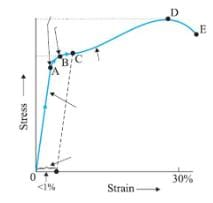Detailed Solution for Test: Mechanical Properties of Solids - Question 29

Hooke’s law: a law stating that the strain in a solid is proportional to the applied stress within the elastic limit of that solid.
In the OA line Hooke’s law is valid because stress is directly proportional to strain.

Test: Mechanical Properties of Solids - Question 30

You hang a flood lamp from the end of a vertical steel wire. The flood lamp stretches the wire 0.18 mm and the stress is proportional to the strain. How much would it have stretched for a copper wire of the original length and diameter?

## Physics Class 11

127 videos|464 docs|210 tests
 Use Code STAYHOME200 and get INR 200 additional OFF Use Coupon Code
Information about Test: Mechanical Properties of Solids Page
In this test you can find the Exam questions for Test: Mechanical Properties of Solids solved & explained in the simplest way possible. Besides giving Questions and answers for Test: Mechanical Properties of Solids, EduRev gives you an ample number of Online tests for practice

## Physics Class 11

127 videos|464 docs|210 tests

### How to Prepare for NEET

Read our guide to prepare for NEET which is created by Toppers & the best Teachers